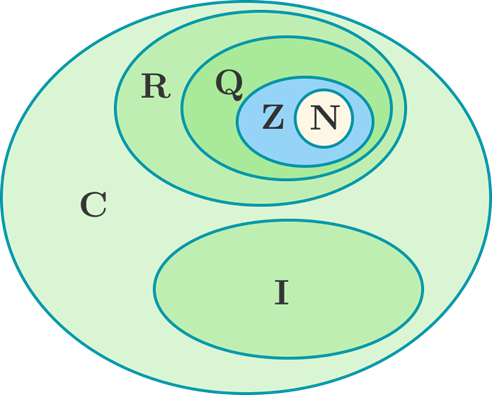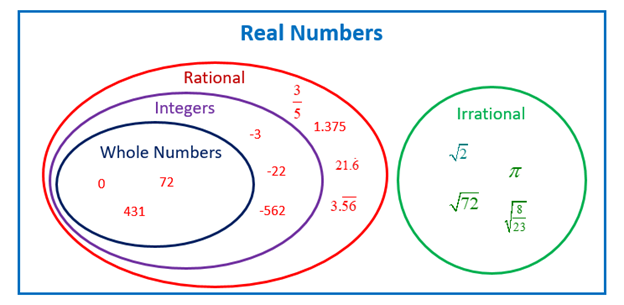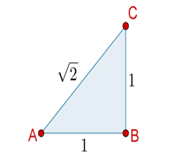Mathematics

# Irrational Numbers

144 views

 1 Introduction 2 History 3 Properties of Irrational Numbers 4 Proof of Irrational Number 6 Solved Examples 7 Practice Questions 8 FAQs

24 September 2020

## Introduction

Irrational Numbers are those set of numbers that can’t be expressed in the form of $$\frac{p}{q}$$ where p and q are integers and $$q≠0.$$ This is in contrast with Rational Numbers, which can be expressed as p/q. This takes us to the crispest definition of Irrational Numbers, which states that it is the set of real numbers that aren’t Rational. There is a very interesting legend behind the development of Irrational Numbers, which Pythagoras tried to drown initially as it was against the nature of mathematics.Irrational Numbers are usually represented by R\Q which also denotes R-Q.

## HistoryThe first man to recognize the existence of irrational numbers might have died for his discovery. Hippassus of Metapontum was an ancient Greek philosopher of the Pythagorean school of thought. Supposedly, he tried to use his teacher's famous theorem

$$a^2+b^2= c^2$$

to find the length of the diagonal of a unit square. This revealed that a square's sides are incommensurable with its diagonal and that this length cannot be expressed as the ratio of two integers. The other Pythagoreans believed dogmatically that only positive rational numbers could exist. They were so horrified by the idea of incommensurability that they threw Hippassus overboard on a sea voyage and vowed to keep the existence of irrational numbers an official secret of their sect. However, there are good reasons to believe Hippassus's demise is merely an apocryphal myth. Historical documents referencing the incident are both sparse and written 800 years after the time of Pythagoras and Hippassus. It wasn't until approximately 300 years after Hippassus's time that Euclid would give his proof for the irrationality of $$\sqrt2.$$

The Pythagoreans had likely manually measured the diagonal of a unit square. However, they would have regarded such a measurement as an approximation close to a precise rational number that gave the true length of the diagonal. Before Hippassus, Pythagoreans had no reason to suspect that there were real numbers that in principle, not merely in practice, could not be measured or counted to. Numbers were the spiritual basis of their philosophy and religion for the Pythagoreans. Cosmology, physics, ethics, and spirituality were predicated on the premise that "all is number." They believed that all things--the number of stars in the sky, the pitches of musical scales, and the qualities of virtue--could all be described by and apprehended through rational numbers.

## Properties of Irrational Numbers

• When we add an irrational number and a rational number, we get an irrational number. To see why this is true, suppose x is irrational, y is rational, and the sum $$x+y$$ is a rational number z. Then we have $$x=z−y,$$ and since the difference of two rational numbers is rational, this implies x is rational. This is a contradiction since x is irrational. Therefore, the sum $$x+y$$ must be irrational
• Multiplication of any irrational number with any nonzero rational number results in an irrational number. We argue as above to show that if $$xy=z$$ is rational, then $$x = \frac{z}{y}$$ is rational, contradicting the assumption that x is irrational. Therefore, the product xy must be irrational.
• The least common multiple (LCM) of any two irrational numbers may or may not exist.
• The sum or the product of two irrational numbers may be rational; For example,

$$\sqrt{2} \times \sqrt{2} = 2.$$

Here, $$\sqrt{2}$$ is an irrational number. If it is multiplied twice, then the final product obtained is a rational number. (i.e) 2.

Therefore, unlike the set of rational numbers, irrational numbers are not closed under multiplication.

## Proof of an Irrational number

We have been discussing the proof that led to a whole new way of looking at Mathematics for long so it is time that we see how it goes.

To Prove:

$$\sqrt{2}$$ is an irrational number.

Suppose, $$\sqrt{2}$$ is a rational number. Then, by the definition of rational numbers, it can be written that,

$$\sqrt{2}=\frac{p}{q} \qquad \dots(1)$$

Where p and q are co-prime integers and $$q ≠ 0$$ (Co-primes are those numbers whose common factor is 1).

Squaring both the sides of equation (1), we have

\begin{align}2 &= p^2/q^2\\⇒ p^2 &= 2 * q^2\qquad \dots(2)\end{align}

From the theorem, that states “Given p is a prime number and a2 is divisible by p, (where a is any positive integer), then it can be concluded that p also divides a”, if 2 is a prime factor of p2, then 2 is also a prime factor of p.

So, $$p = 2 × c,$$ where c is an integer.

Substituting this value of p in equation (3), we have

\begin{align}(2c)^2 &= 2q^2\\⇒ q^2 &= 2c^2\end{align}

This implies that 2 is a prime factor of q2 also. Again from the theorem, it can be said that 2 is also a prime factor of q.

According to the initial assumption, p and q are co-primes but the result obtained above contradicts this assumption as p and q have 2 as a common prime factor other than 1. This contradiction arose due to the incorrect assumption that $$\sqrt{2}$$ is rational.

So, $$\sqrt{2}$$ is irrational.

## Examples of Irrational Numbers1. The hypotenuse of a right triangle with base sides of length 1 has length $$\sqrt{2}$$ which is irrational. More generally, $$\sqrt{D}$$ is irrational for any integer D that is not a perfect square.

2. The ratio π of the circumference of a circle to its diameter is irrational.

3. The base e of the natural logarithm is irrational.

4. The number φ (Golden Ratio) is an irrational number.\begin{align} \pi=3.1415926535\\\\e=2.7182818284\\\\\sqrt{2}=1.4142135623\end{align}

## Solved Examples

 Example 1

\begin{align}\pi\times e,\pi\end{align}

Find the lowest common multiple (L.C.M.) of the given above two numbers?

1. LCM does not exist
2. e
3. $$\pi$$
4. $$e * π$$
5. None of the above

Solution:

One might be tempted to select the answer as d) $$e * π$$ but that would be the wrong answer.

If LCM of a and b is L, then $$L=a \times n=b \times m$$ where n and m are integers.

Now if LCM of $$π \times e$$ and $$π$$ exists then $$e=\frac{m}{n}.$$ But this can't be true as e is an irrational number and m/n is rational. Our assumption was wrong therefore LCM of $$π \times e$$
and $$\pi$$ does not exist.

 Example 2

$$−6\pi, \pi$$

Find the lowest common multiple (LCM) of the two numbers above.

1. $$-6π$$
2. $$6π$$
3. $$π$$
4. $$-π$$
5. None of the above
6. LCM doesn’t exist

Unlike the previous example, the division of these two numbers is in fact a rational number so LCM surely can exist. LCM is always positive so here we ignore the negative sign. $$π$$ is a common factor between both the numbers $$π,\,6π$$

The L.C.M of 1 and 6 is 6.

From the above statements, we can say the L.C.M is 6π

## Practice Questions

1. Classify each number as a rational or an irrational number.

1. $$\sqrt{4}$$
2. $$27.5$$
3. $$\sqrt{37}$$
4. $$1/9$$
5. $$0.09$$
6. $$0.895210351$$
7. $$0.285714$$
8. $$\sqrt{64}$$
9. $$\sqrt{2}$$
10. $$11.5$$

2. All integers are ______ numbers.

• Rational
• Irrational

3. I have a number that cannot be expressed in the form $$𝑎𝑏$$ where $$𝑎,𝑏∈ℤ,$$ and $$𝑏≠0.$$ Is this number rational or irrational?

• Irrational number
• Rational number

4. Without using a calculator, order the numbers $$−4𝜋,−4√3,𝜋,12,$$ and $$−3 \sqrt{4}$$ from smallest to largest.

• $$\text{A}-4 \pi,-3 \sqrt{4},-4 \sqrt{3}, \pi, 12$$
• $$\text{B}-4 \pi,-4 \sqrt{3},-3 \sqrt{4}, \pi, 12$$
• $$\text{C}-4 \pi,-4 \sqrt{3},-3 \sqrt{4}, 12, \pi$$
• $$\text{D} {12}, \pi,-4 \sqrt{3},-3 \sqrt{4},-4 \pi$$
• $$\text{E}-3 \sqrt{4},-4 \sqrt{3}, \pi, 12,-4 \pi$$

5. On the number line, which arrow represents the value of $$𝜋$$?• b
• c
• A

6. What is $$ℚ−ℚ′$$?

• $$ℚ$$
• {0}
• $$ℤ$$

7. A square of side length $$𝑥 \rm{cm}$$ has an area of $$280 \text{ cm}^2.$$ Which of the following is true about $$𝑥$$?

• It is an integer number.
• It is an irrational number.
• It is a negative number.
• It is a natural number.
• It is a rational number.

## What is the difference between rational and irrational numbers?

Difference Between Rational and Irrational Numbers

 Rational Number Irrational Numbers ● It is expressed as p/q, where both p and q are integers. ● It is impossible to express irrational numbers as simple fractions or as a ratio of two integers ● It includes perfect squares ● It includes surds ● The decimal expansion for rational number executes finite or recurring decimals ● Here, non-terminating and non-recurring decimals are executed

## How to identify Irrational Numbers?

• Irrational Numbers are real numbers that aren’t Rational i.e. any number that cannot be expressed as p/q, where p and q are integers is irrational.

• The previous point is helpful for identifying irrational numbers from fractions.

• When we talk about Decimal representation of numbers, any number that has a terminating decimal representation or non-terminating repeating decimal representation is called Rational and any number with non-terminating non-repeating decimal representation is Irrational

For Example, $$1.6666…$$ is Rational Number

$$3.1484742326…$$ is Irrational Number

## What are the five examples of irrational numbers?

There are many irrational numbers that cannot be written in simplified form. Some of the examples are: $$\sqrt{8},\; \sqrt{11},\;\sqrt{50},$$  Euler’s Number $$e = 2.718281$$, Golden ratio, $$φ= 1.618034.$$

## Is an irrational number a real number?

Yes, an irrational number is a real number and not a complex number, because it is possible to represent these numbers in the number line.

Related Articles
GIVE YOUR CHILD THE CUEMATH EDGE
Access Personalised Math learning through interactive worksheets, gamified concepts and grade-wise courses